# 机器学习中对矩阵的迹（trace）求导的一些操作

机器学习中经常涉及到一些优化工作，优化时又涉及矩阵、向量的Frobenius 范数（Frobenius-Norm），这个F范数又可以转化成矩阵自乘求迹trace的形式，然后对trace求导。这块没学过矩阵论的话，有时候经常会感觉到困惑。所以这里找到一篇文章，有助于理解这块的内容。同时我也在一些地方留了笔记，更有助于理解。

Errata:

$Y$是n维列向量，$\theta$是n维列向量，$X$是mxn维矩阵：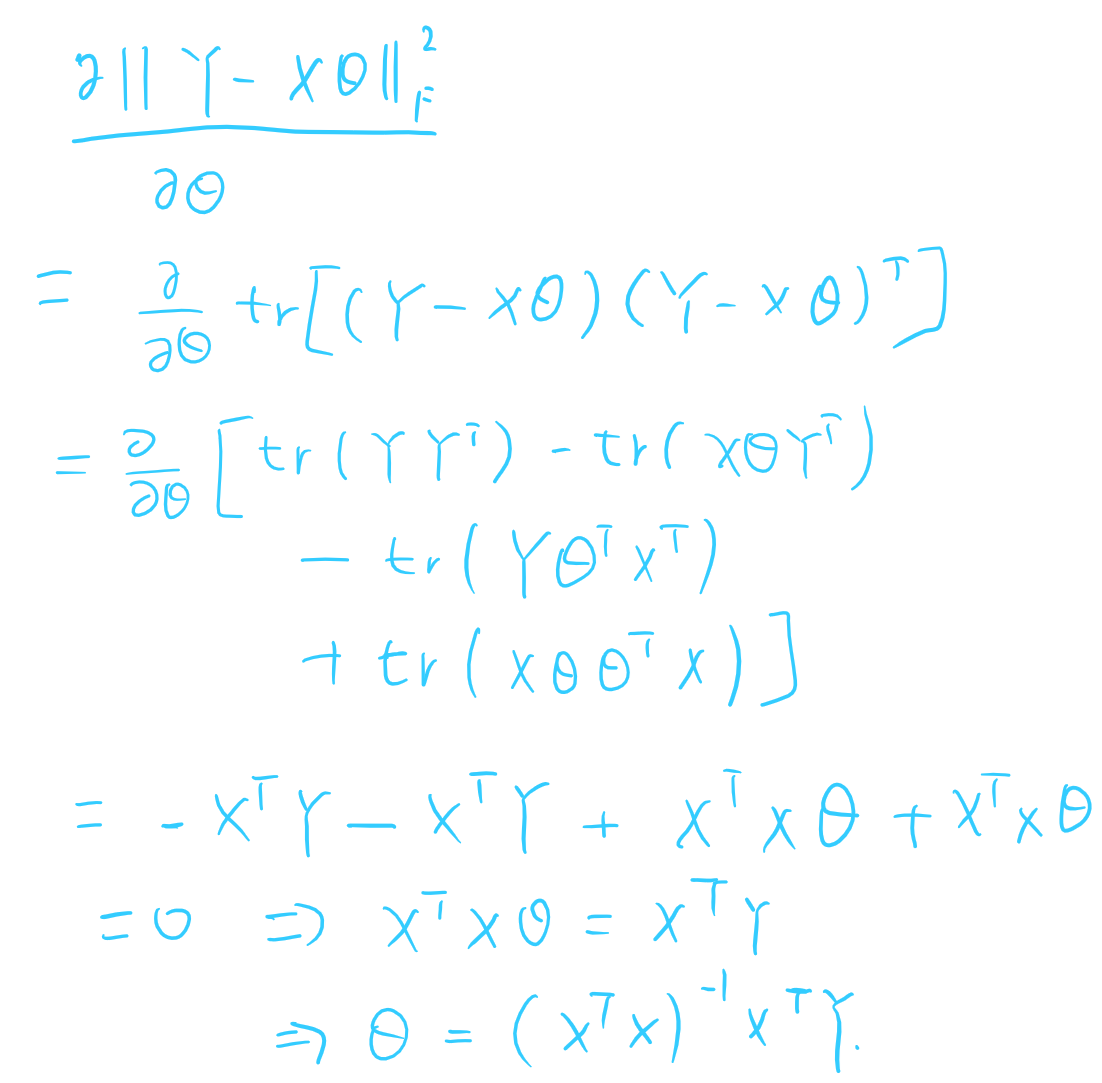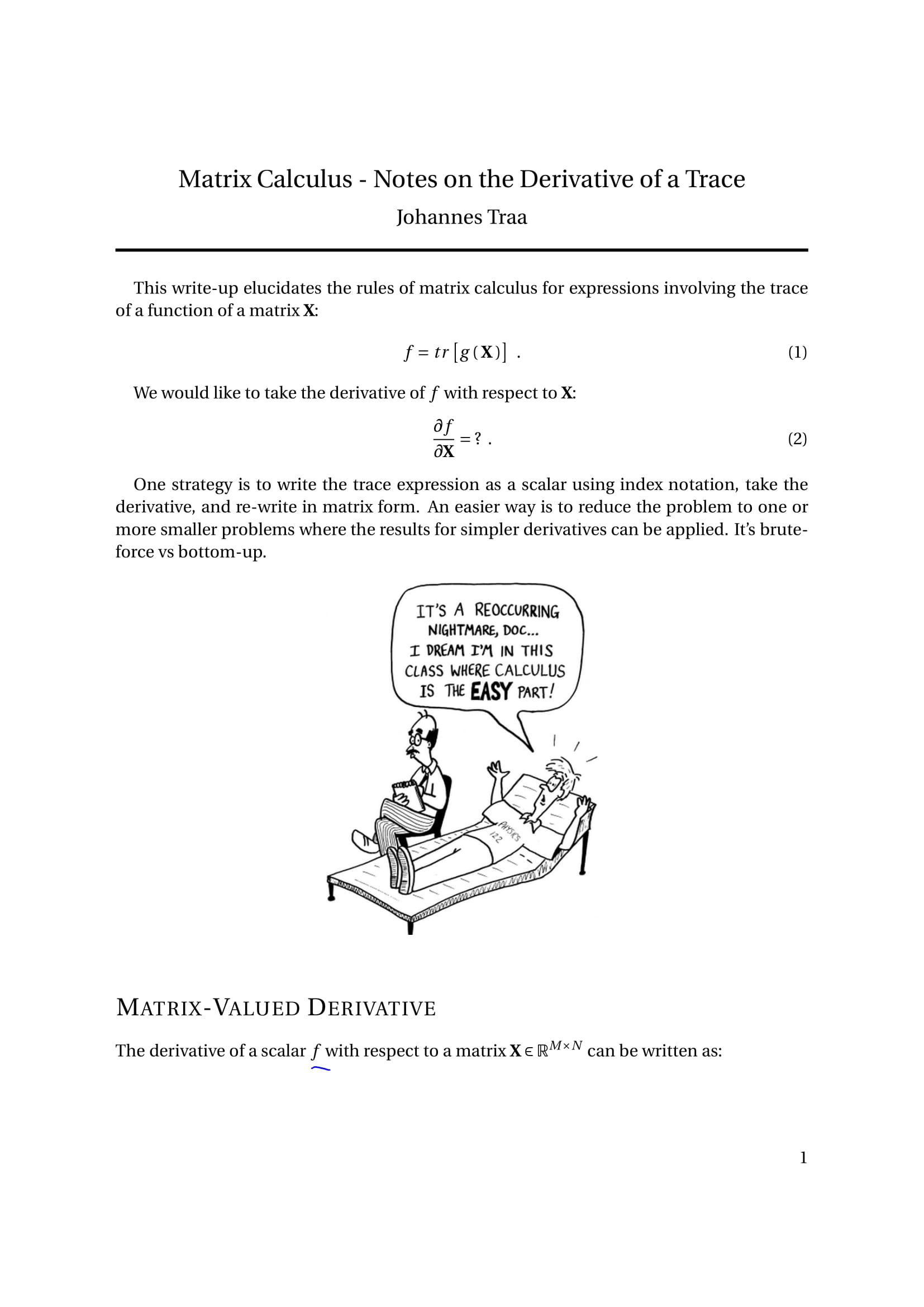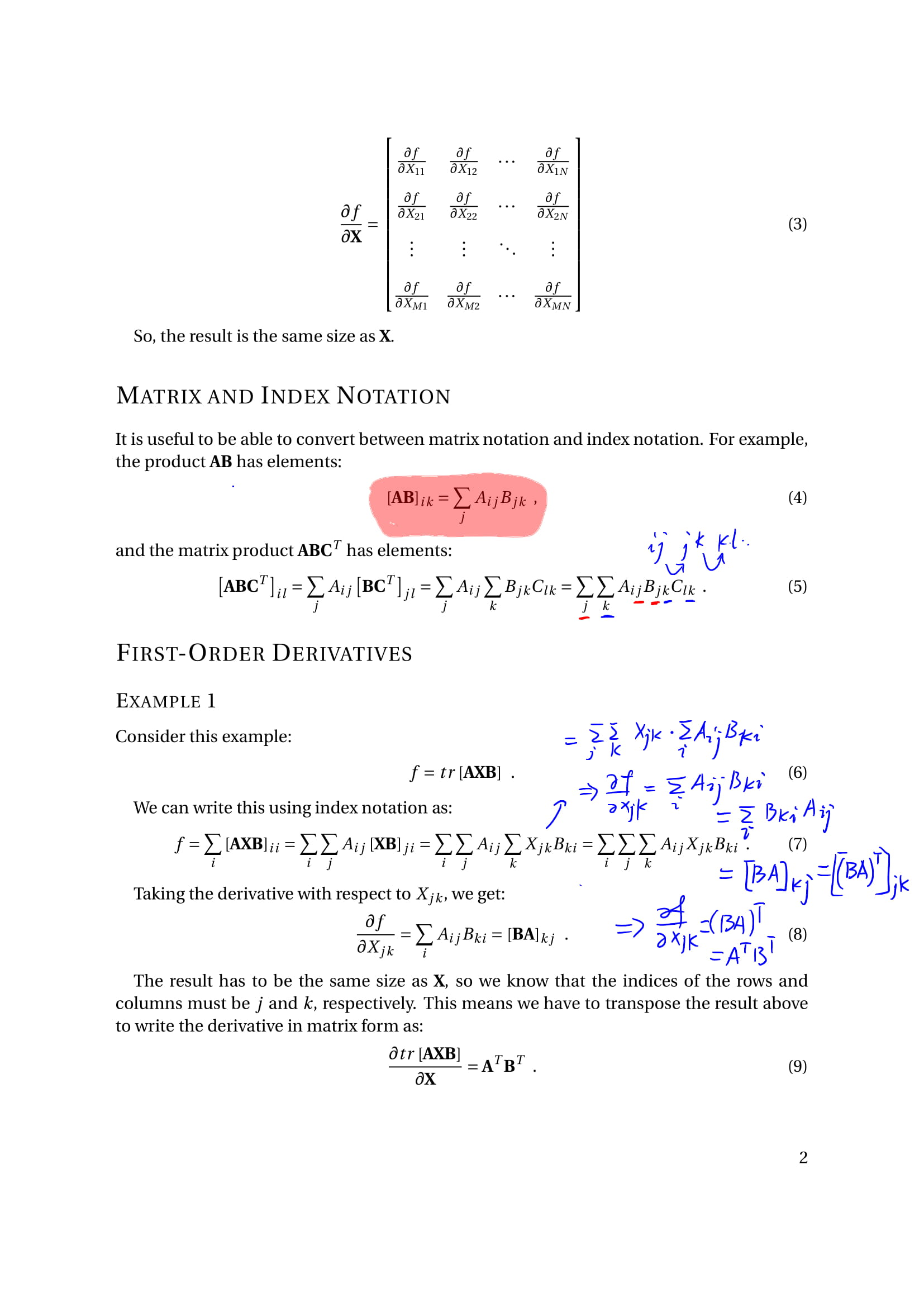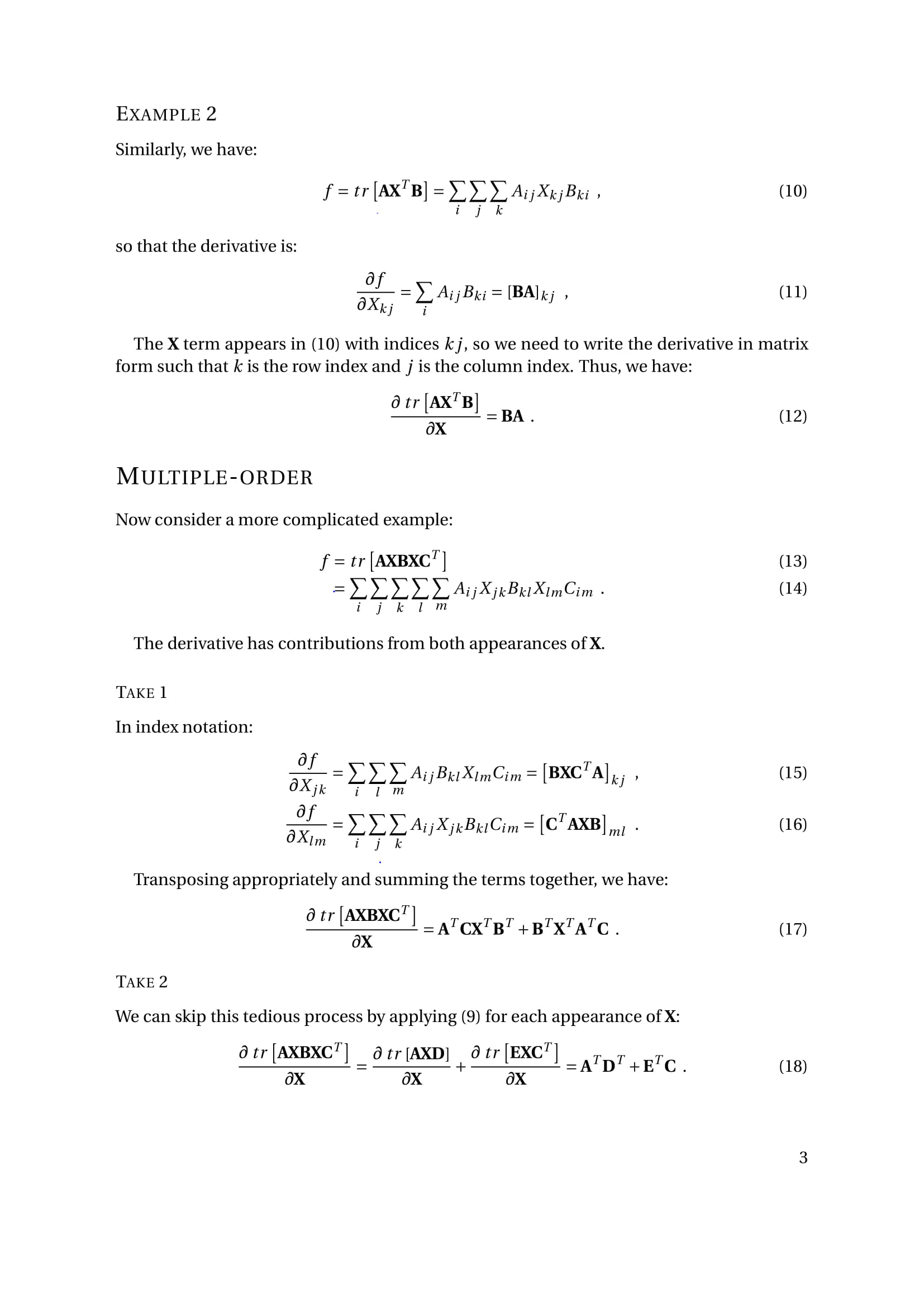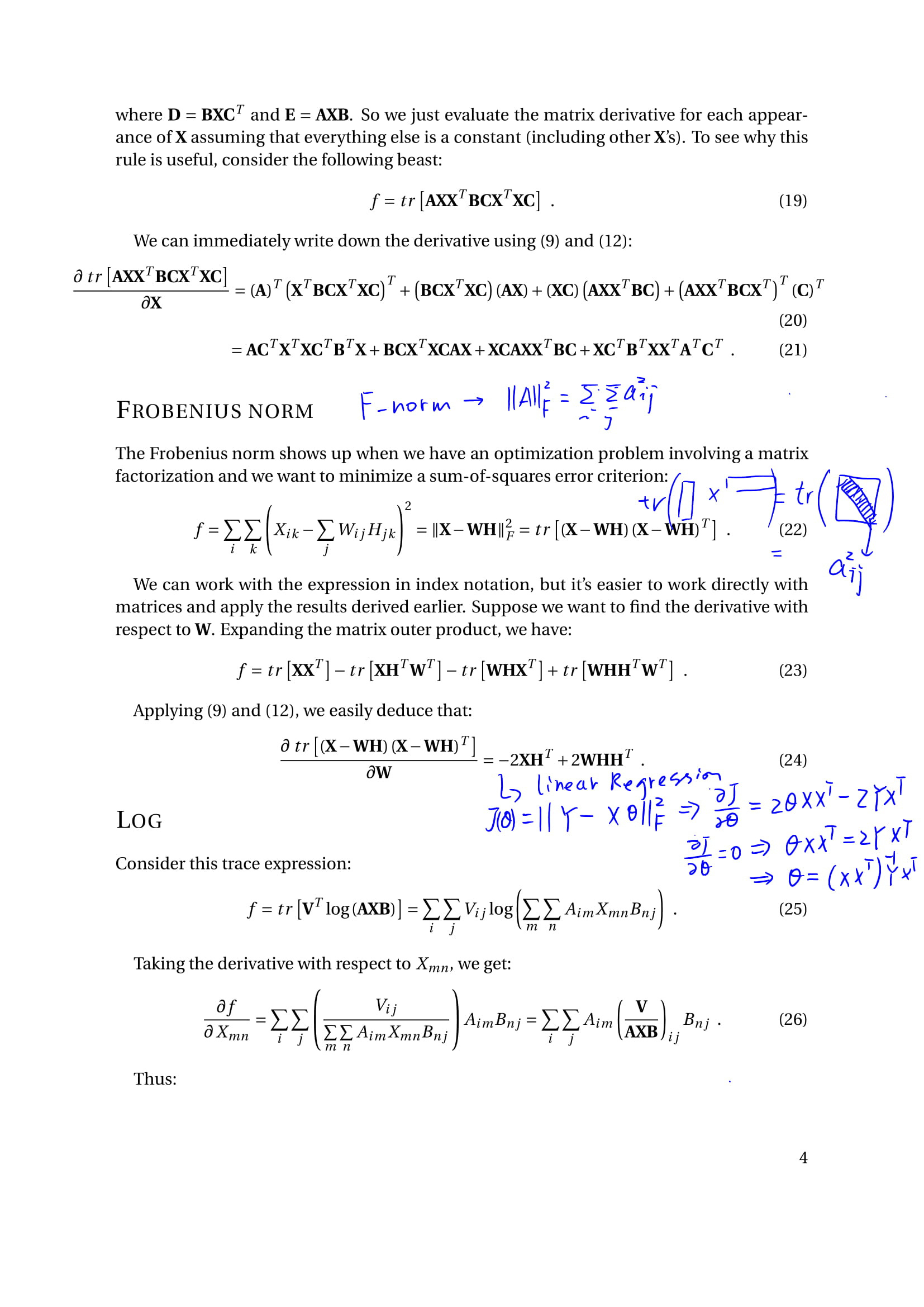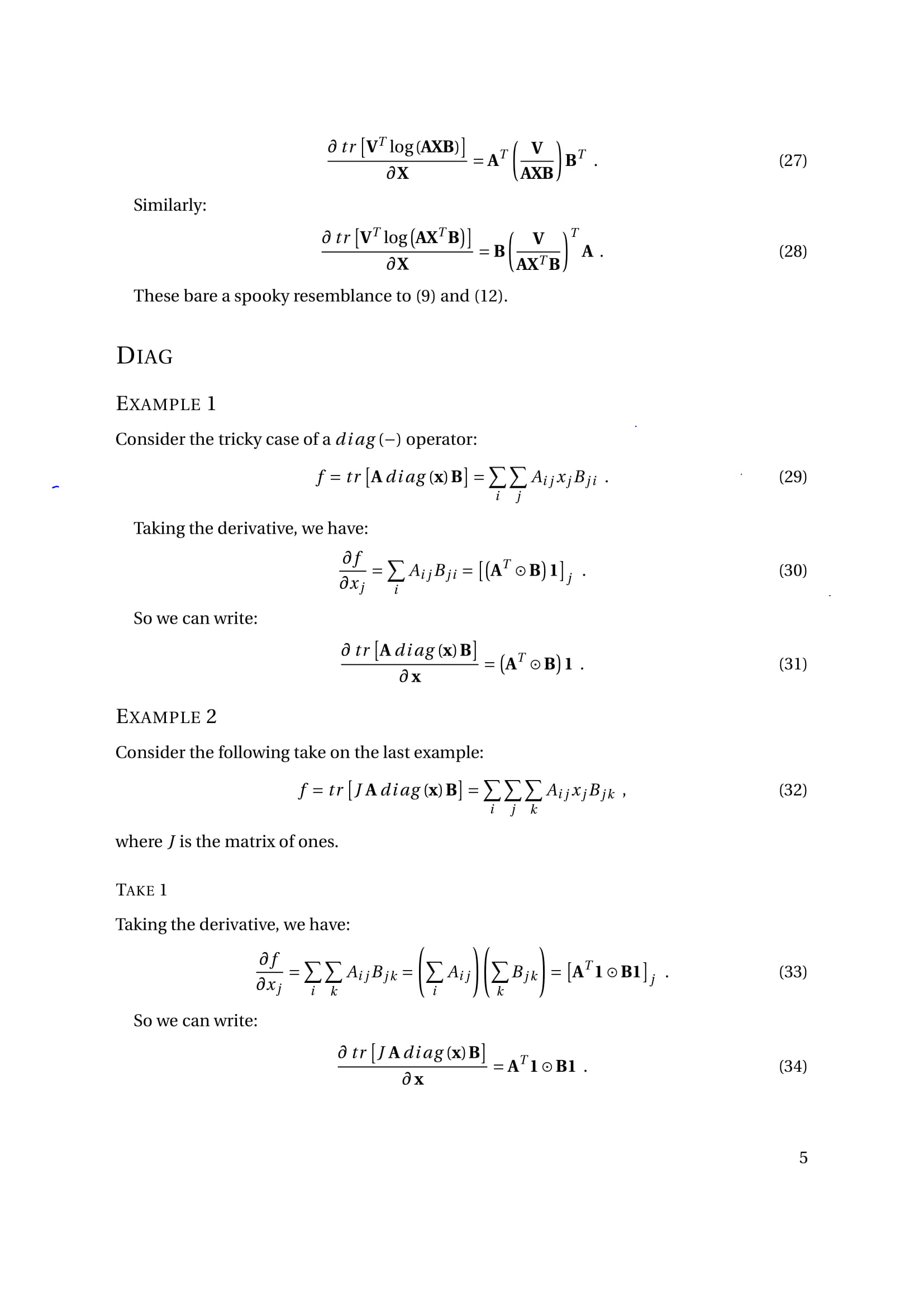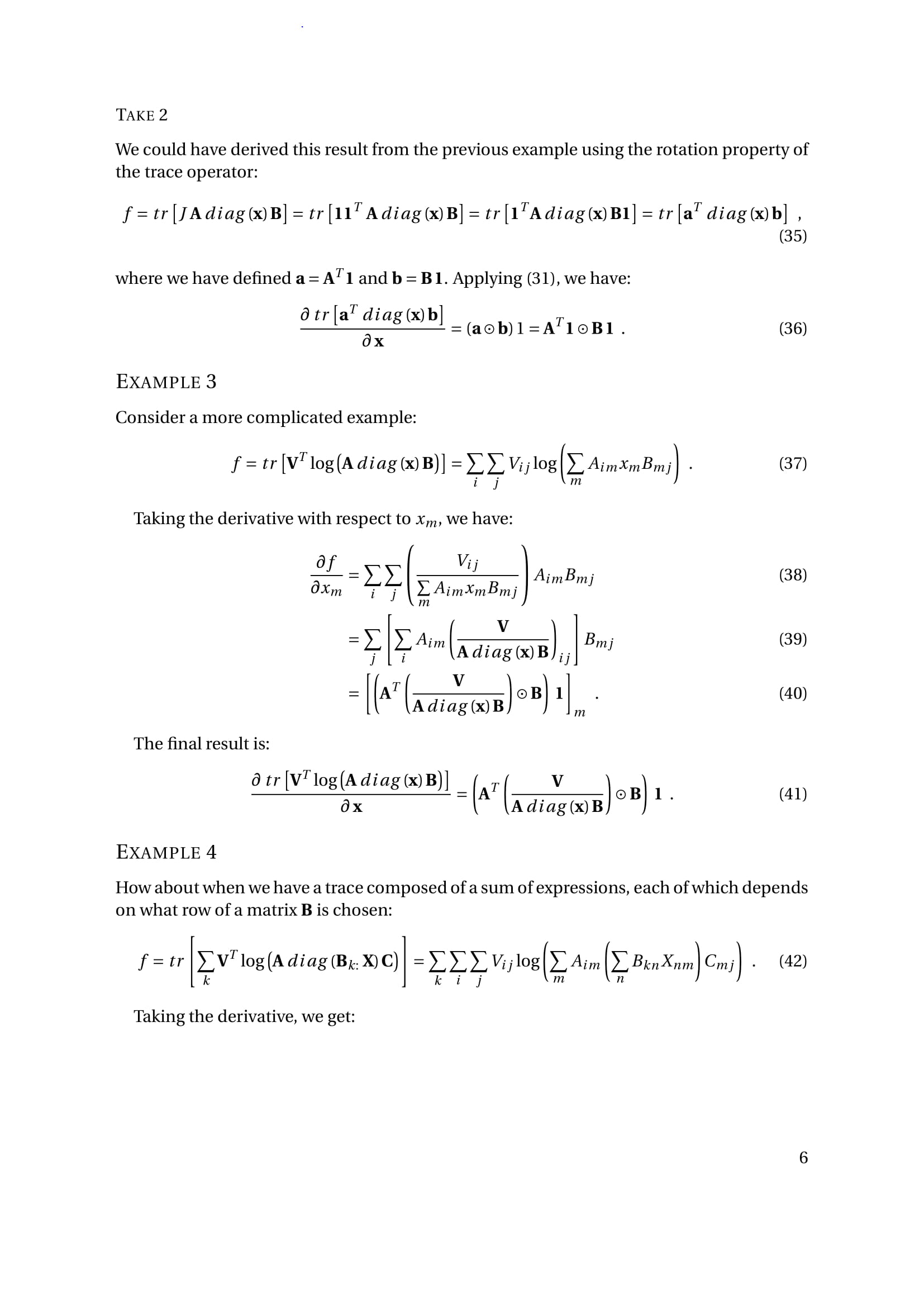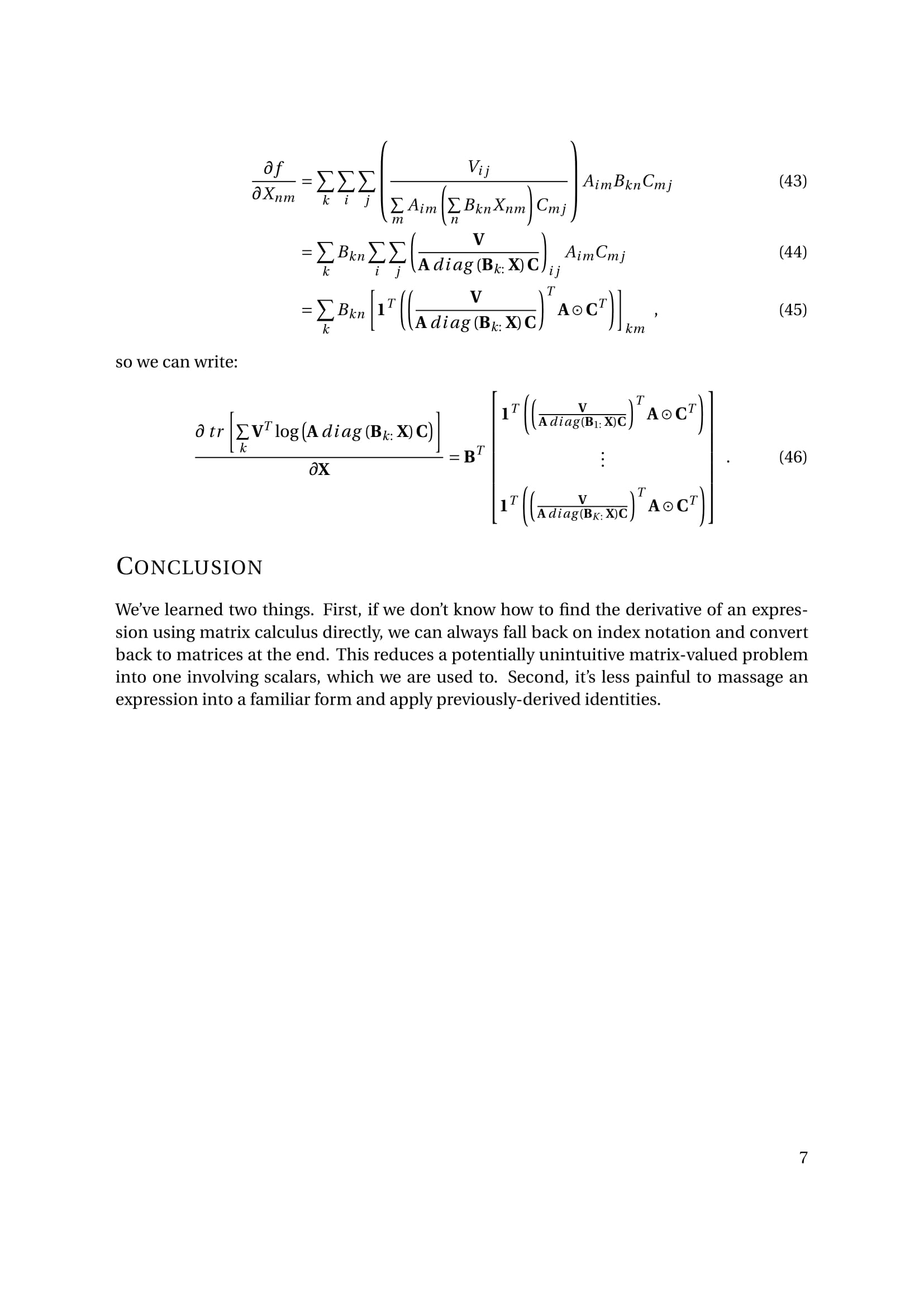（17），这个搞定，很多线性情况都可以解决了。

（9）：
$\frac{\partial{tr(AXB)}}{\partial{X}} = (BA)^{T}=A^TB^T$
（11）：
$\frac{\partial{tr(AX^TB)}}{\partial{X}} = BA$